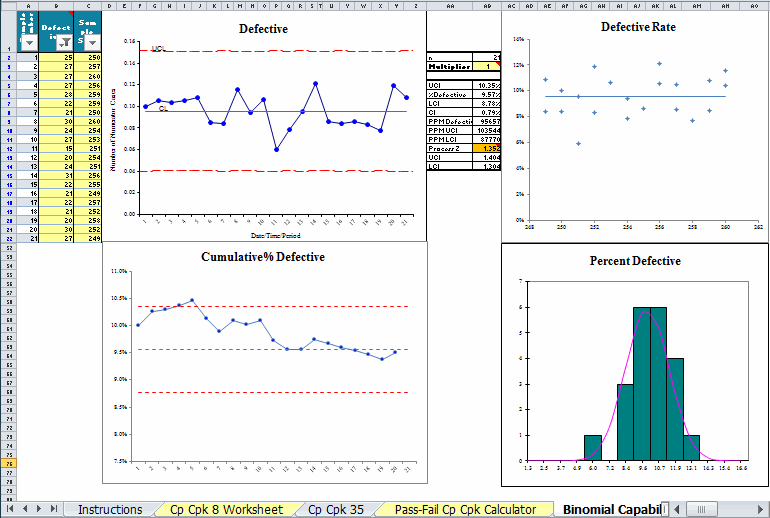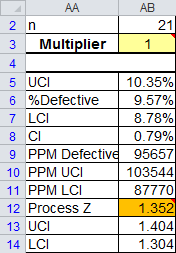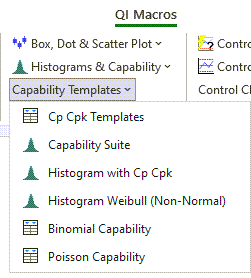# Need to do Binomial Capability Analysis But Don't Know How?

## QI Macros will do the calculations and analysis for you right in Excel!

A Binomial test only has two outcomes: defective or non-defective.

### Example of QI Macros Binomial Capability Template### The Binomial Capability Template Consists of these Charts and Calculations:

• p chart of fraction defective to determine if the process is stable or not.
• chart of cumulative % defective
• the defective rate chart to determine if your data is binomial.
• the percent defective chart
• Process Z measures the capability of your process. The desired level is 2 or greater. For any value less than 2, cell AB12 will <strong> turn orange</strong> to indicate lack of capability.

Tip: If your data is unstable and/or does not follow the Binomial Distribution, the calculated results may not be reliable.

### Example of Binomial Analysis Using the Output Shown Above

• The p chart is in control so capability analysis is possible. (Out of control data, as mentioned above, is not recommended)
• The Cumulative % Defective chart is also pretty stable.
• The Defective Rate chart shows points evenly distributed on either side of the average, so distribution is Binomial.
• The Process Z score is 1.352. Since it is less than 2, the template flags it with orange to indicate the lack of capability.
• If the Upper Confidence Interval (UCI) for percent defective (cell AB:3 of 10.35%) is greater than the target percent defective (cell AB:6 9.57) , then you cannot be 95% confident that the process is capable.You can find this template by selecting QI Macros menu > Capability Templates > Binomial Capability.